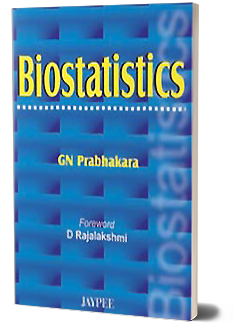Biostatistics

by GN Prabhakara

The book “Biostatistics” provides a simple, systematic and useful reference to trainers in Biostatistics and trainers of Biostatistics in BIOMEDICAL field who are involved in human beings in their health and disease. The book gives vivid picture of learning by doing of Statistical Techniques. Author has distributed his ideas in SEVEN different sections to cover Prologue, Basics of Statistics, Understanding Statistics, Specific Statistical Techniques, Present area of interest, Appendix and Yellow Pages of Bio Statistics. Section 1 is dealt with first chapter giving modest definitions of terminologists used in the text book. Readers are allowed to study and learn from problems. Glossary of the chapter follows. Section 2 deals with 3 chapters giving Basics of Statistics through Data collection, Data tabulation and Data presentations. Chapter 2 gives regular and routine data collecting systems. Chapter 3 gives different tables made use of in tabulation. Chapter 4 gives illustrated views of charts and diagrams. Readers are allowed to study and learn from problems in each chapter. Glossary follows in each chapter. Section 3 deals with 5 chapters that are required for our understanding of statistics. Chapter 5 introduces and describes three commonly used measures of central tendency mean, median and mode. Chapter 6 describes with illustration, the measures of variability that are commonly used in biostatistics. Range, Mean Deviation, Standard Deviation, Co efficient of Variation and Quartile Deviation are illustrated. Chapter 7 details Confidence Intervals when we have true mean, true proportion, two means, two proportions and when we have independent values or paired values. Chapter 8 details Normal Curve commonly used in Biostatistics as a statistical property. Chapter 9 outlines Decision Making process in biostatistics with the help of values of means and proportions. Readers are allowed to study and learn from problems in each chapter. Glossary follows in each chapter. Section 4 deals on specific statistical techniques through eleven chapters. Chapter 10 gives introduction and details different types of sampling like simple random sampling, stratified sampling, systematic sampling, multistage sampling, cluster sampling and area sampling. Chapter 11 effectively works out the determination of sample size in scientific studies in biostatistics. Chapter 12 brings out standard error of the mean and proportion, standard error of difference between 2 means and 2 proportions and normal deviate that are considered as basic statistical significant tests. Chapters 13 and 14 bring out the probabilities of occurrence in normal situation and in rare situation through binomial distribution and Poisson distribution respectively. Chapter 15 details very common but routinely done chi square test that is used for goodness of fit and for the measure of the degree of association. Chapter 16 details small sample statistical significant test called student-t test which is used in a sample size of below 30 in biostatistics. Chapter 17 describes present dictum of multi variate analysis popularly known as ANOVA. Chapters 18, 19 and 20 gives vivid picture of correlation test, regression test and rank correlation test that are commonly used in medical research, health science research, public health research, food research and genetic research. Section 5 is depicted with 4 chapters that describe ‘present area of interest’. Chapter 21 deals with population statistics which include morbidity, mortality and fertility. Chapter 22 details life table for probability estimation of survival or death. Chapter 23 deals with multi dimensional approach to medical or health problems wherein measures to be taken based on decision arrived in such problems are discussed. Chapter-24 deals with other biostatical techniques with particular reference with multiple correlation, Meta analysis and cluster analysis. Section-6 is appended with requirements of biostatistics. Square of numbers, square, square root, reciprocals, random numbers and major statistical tables are referred in the statistical inferences. Section 7 is compiled with commonly used formulae by the author in yellow pages as ready references. It also has expansions of abbreviations used in the text.

[ Please select above dropdown to change Section ]

Chapters of the selected section will be displayed in the chapter section below.

Book Detail

Author Author's Affiliation
1. Prabhakara GN SDM College of Medical Sciences and Hospital, Manjushree Nagar, Sattur, Dharwad, Karnataka, India
Medical Arts Photography and Modelling, SDM College of Medical Sciences and Hospital, Manjushree Nagara, Sattur, Dharwad, Karnataka
Karnataka Institute of Medical Sciences, Hubli, India
SDM Medical College, Dharwad, Karnataka, India
ISBN 9788180616594
Speciality Biostatistics and Research Methodology
DOI 10.5005/jp/books/10102
Edition 1/e
Publishing Year 2006
Pages 356
Book Type Textbook

Related Books

• How to Write the Thesis and Thesis Protocol: A Primer for Medical, Dental, and Nursing Courses

Piyush Gupta, Dheeraj Shah

• Quantitative Research Methods in Health Sciences

Rajendra Karkee

R Sudha

• Nursing Research

Renjith Augustine

Rajesh Kumar

• Synopsis Dissertation and Research to PG Students

GN Prabhakara